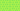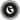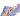PricingResources

# How to Calculate Future ValueWritten by

As a business owner, when you invest, you are looking to grow your wealth. But is the investment you’re about to make the right investment? Will it actually pay off in the long run and yield the desired profit? Calculating future value will help you to identify whether or not an investment is justified. In this article, we look at what future value is and how to calculate future value of annuity, with some helpful examples.

## What is future value?

Within finance and accounting, future value, commonly abbreviated to FV, is the value of a current asset in the future, based on an assumed rate of interest. This could be anything from stocks and bonds to savings and annuities. Essentially, it determines the effect time has on money - working to predict what today's investment could be worth years down the line.

If you know how to work out the future value, it will help you to make smart investment decisions. It is, however, important to understand that future value is an estimate; it’s not definitive. External economic factors, such as inflation and fluctuating interest rates, will affect the future value and may lead to an inaccurate result.

## Calculating future value of annuity

There are two ways of calculating the future value of annuity. It depends on if you are using compound interest or simple interest. What’s the difference between the two? Well, simple interest is calculated on the principal, or original, amount of a loan or deposit, whereas compound interest is calculated on the principal amount, as well as the interest that accumulates on it every period.

## Calculating future value using simple interest

To calculate the future value of an annuity earning simple interest, you first need the present value of the investment, the interest rate (R) and the number of interest-compounding years that will occur during the time period you’re calculating for (T).

There are a couple of different versions of the future value formula, but, in its most basic form, the equation is:

Future value = present value x [1 + (interest rate x number of years)]

If we break it down, that’s:

Here’s an example:

Suppose a £1,000 investment is held for 3 years in a savings account with a 10% simple interest paid annually. What would the future value of the investment be?

FV = 1000 x [1 + (0.10 x 3)]

FV = £1,300

## Calculating future value using compound interest

If you need to calculate the future value of an investment earning compound interest, the formula is slightly different. As opposed to simple interest, here, the rate is applied to each period’s cumulative account balance.

Here’s an example:

We can use the same example as above. So, if £1,000 is invested for 3 years in a savings account with a 10% compounding interest rate, what would the future value of the investment be?

FV = 1000 x [(1 + 0.10)3]

FV =  £1,331.00

Not feeling up to using the future value annuity formula? Online future value calculators are also available to help you quickly find out the FV of your investment. However, you must ensure the source is reliable. After all, we want an accurate result to determine the feasibility of the investment!

#### We can help

GoCardless helps you automate payment collection, cutting down on the amount of admin your team needs to deal with when chasing invoices. Find out how GoCardless can help you with ad hoc payments or recurring payments.Contact sales

Sales

Support